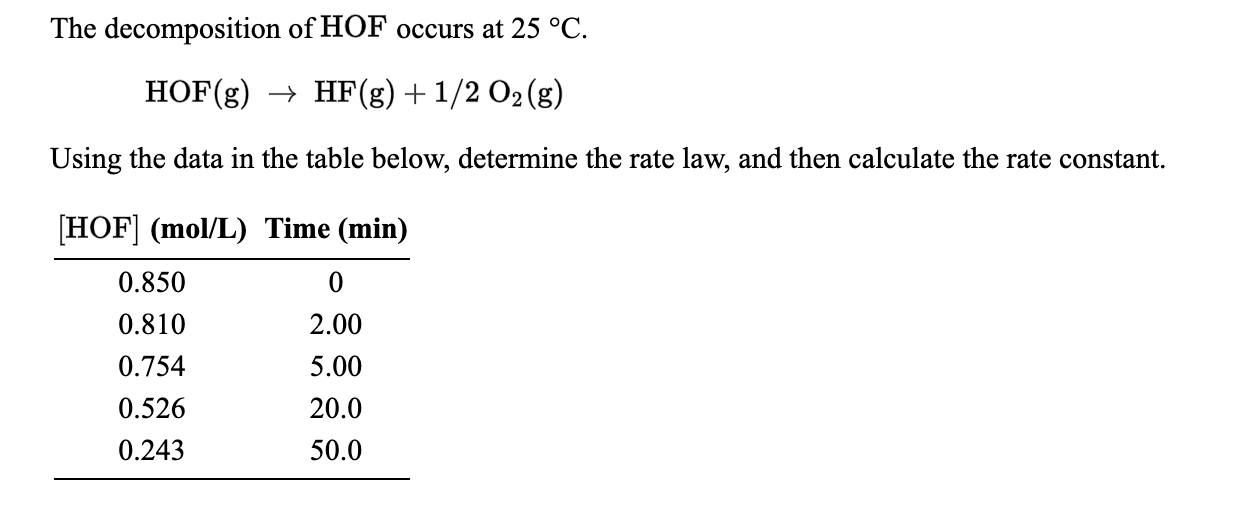# The decomposition of HOF occurs at 25 °C HF(g) 1/2 O2(g) HOF(g) Using the data in the table below, determine the rate law, and then calculate the rate constant HOF (mol/L) Time (min) 0.850 0 0.810 2.00 0.754 5.00 20.0 0.526 0.243 50.0

Question

What is the rate? and what is the rate constant?help_outlineImage TranscriptioncloseThe decomposition of HOF occurs at 25 °C HF(g) 1/2 O2(g) HOF(g) Using the data in the table below, determine the rate law, and then calculate the rate constant HOF (mol/L) Time (min) 0.850 0 0.810 2.00 0.754 5.00 20.0 0.526 0.243 50.0 fullscreen

### Want to see the step-by-step answer?